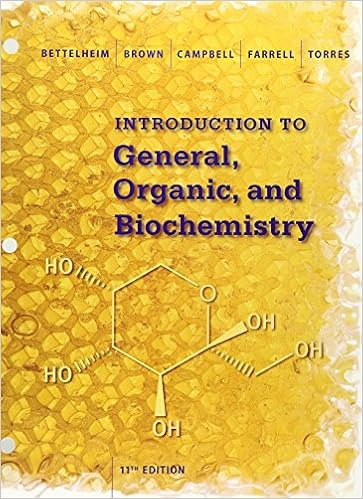# Which pair of species is isoelectronic a ne and na b

• Test Prep
• 9
• 100% (1) 1 out of 1 people found this document helpful

This preview shows page 7 - 9 out of 9 pages.

Exam 3 1307 v-5D.pdf

26 )   Cold packs , whose temperatures are lowered when ammonium nitrate dissolves in water , are carried by athletic trainers when transporting ice is not possible . The dissolution reaction can be depicted as follows : NH 4 NO 3 ( s ) + heat H 2 O NH 4 NO 3 ( aq ) Which of the following is true of this reaction ?
A ) Δ H < 0 , process is exothermic
B ) Δ H = 0 , since only a physical change takes place
C ) Δ H > 0 , process is endothermic
D ) Δ H > 0 , process is exothermic
E ) Δ H < 0 , process is endothermic
27 )   Which pair of species is isoelectronic ?
2 -   28 ) The FM station KDUL broadcasts music at 99.1 MHz . Find the wavelength of these waves .
28 )   The FM station KDUL broadcasts music at 99.1 MHz . Find the wavelength of these waves .
29 )   In chemistry , a theory :
A ) is the measurement that is closest to the " true " value .
B ) can not be proven or disproven .
C ) explains a body of facts based upon hypotheses that have been proven to be true .
D ) is required before performing an experiment .
E ) is a possible but unproven explanation for an observation .
30 )   Calcium hydroxide , which reacts with carbon dioxide to form calcium carbonate , was used by the ancient Romans as mortar in stone structures . The reaction for this process is Ca ( OH ) 2 ( s ) + CO 2 ( g ) → CaCO 3 ( s ) + H 2 O ( g ) Δ H = – 69.1 kJ What is the enthalpy change if 3.8 mol of calcium carbonate is formed ?
31 )   Select the gas with the largest root - mean - square molecular speed at 25 ° C
32 )   Which of the following elements has the lowest first ionization energy ?
##### We have textbook solutions for you!
The document you are viewing contains questions related to this textbook.The document you are viewing contains questions related to this textbook.
Chapter 5 / Exercise 5-49
Introduction to General, Organic and Biochemistry
BrownExpert Verified
27) Which pair of species is isoelectronic?A) Ne and Na+B) Ca2+and KrC) Be2+and Mg2+D) H+and HeE) O2-and S
2-28) The FM station KDUL broadcasts music at 99.1 MHz. Find the wavelength of these waves.
m
m29) In chemistry, a theory:
7
##### We have textbook solutions for you!
The document you are viewing contains questions related to this textbook.The document you are viewing contains questions related to this textbook.
Chapter 5 / Exercise 5-49
Introduction to General, Organic and Biochemistry
BrownExpert Verified
30) Calcium hydroxide, which reacts with carbon dioxide to form calcium carbonate, was used by theancient Romans as mortar in stone structures. The reaction for this process is Ca(OH)2(s) + CO2(g) CaCO3(s) + H2O(g) ΔH= –69.1 kJWhat is the enthalpy change if 3.8 mol of calcium carbonate is formed?
31)Select the gas with the largest root-mean-square molecular speed at 25°CA) NH3B) SF6C) COD) H2E) All the gases have the same root-mean-square molecular speed at 25°C.
32) Which of the following elements has the lowestfirst ionization energy?
8
Answer KeyTestname: EXAM 3 1307 V-5B
9
•••